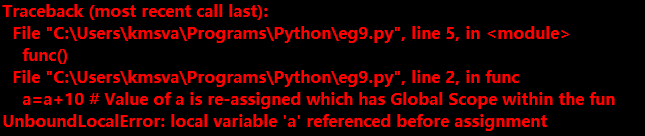# Create a Global Variable in Python

This tutorial is about the creation of a global variable in Python with the usage of the Global Keyword.

The variables which have scope till the end of the program are called Global Variables. Generally, we will use global variables outside the functions. In this tutorial, we need to use them inside the Local Scopes.

See the Python program below:

```#The following Function can access a Global Variable
def func():
print("Inside a Function a =",a)      # a is Global Variable

a=10
func()```

Now see the output of the above program:

`Inside a Function a = 10`

We were just using the value of a in the function in the above code. The scope of a will be Local Scope if it is assigned again in the function. The variables which have Global Scope cannot be referenced in Local Scope. Consider the following example

```def func():
a=11                           # a is assigned to a new value. So, Scope Changed to Local
print("Inside a Function a =",a)  # a has Local Scope
a=10                               # a has Global Scope
func()
print("Ouside a Function a =",a)```

Below is the output:

```Inside a Function a = 11
Outside a Function a = 10```

In the above, the value of an inside function before assignment has Global Scope. So, Outside the Function, variable a has Global Scope.

But, when we redefined the value of the variable a, its scope changed to Local. When a is Referenced in a Local Scope, we may get Unbound Local Error. It states that we can’t define a Global Scoped Variable in a Local Scope. Lets have a look at below example

```def func():
a=a+10   # Value of a is re-assigned which has Global Scope within the function
print("After Adding 10 Value of a is",a)
a=10
func()```

Hence in the above code, a global variable is assigned in local scope which cannot be bounded. So, It leads to UnboundLocalError Exception which can be expressed as follows

The output of the program will give the error that is given below:global Keyword enables us to use a global variable in local scope. Its usage is as follows…

• It should be the FIRST Statement in a function

#### Syntax:

• global variable_name [, variable_name_1, variable_name_2, …]

#### Usage of  ‘global’ Keyword:

```def func():
global a
a=a+10
print("Inside Function")
print("a =",a)
a=10
print("Ouside Function before calling Function a =",a)
fun()
print("Outside Function after calling Function a =",a)```

The output of the above code should be as follows

```Outside Function before calling Function a = 10
Inside Function
a = 20
Outside Function after calling Function a = 20```

Hence, in this way, we can use global keyword to create a global variable in Python. Whatever the changes are done inside the Function are reflected to the value of the variable outside the function. i.e. The Value outside the Function changes permanently if it referenced inside the Local Scope i.e. inside a Function.